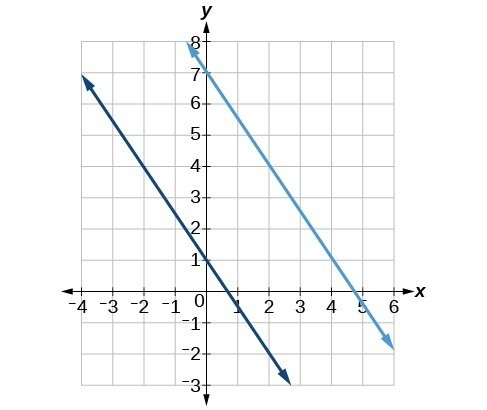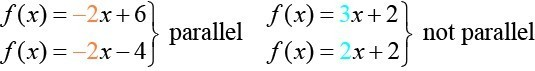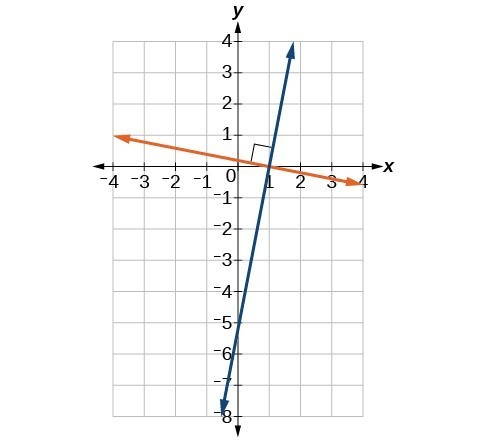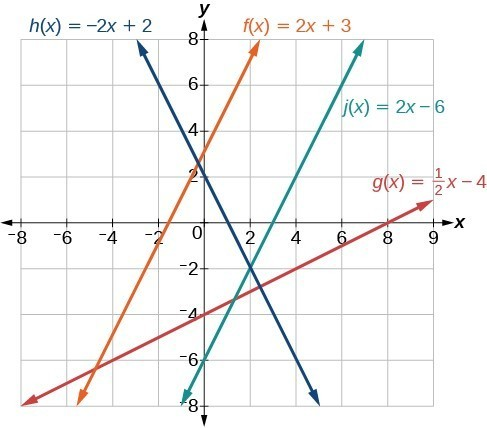## Given the equations of two lines, determine whether their graphs are parallel or perpendicular

The two lines in Figure 18 are parallel lines: they will never intersect. Notice that they have exactly the same steepness, which means their slopes are identical. The only difference between the two lines is the y-intercept. If we shifted one line vertically toward the y-intercept of the other, they would become the same line.Figure 18. Parallel lines.Figure 19.

We can determine from their equations whether two lines are parallel by comparing their slopes. If the slopes are the same and the y-intercepts are different, the lines are parallel. If the slopes are different, the lines are not parallel.

Unlike parallel lines, perpendicular lines do intersect. Their intersection forms a right, or 90-degree, angle. The two lines in Figure 20 are perpendicular.Figure 20. Perpendicular lines.

Perpendicular lines do not have the same slope. The slopes of perpendicular lines are different from one another in a specific way. The slope of one line is the negative reciprocal of the slope of the other line. The product of a number and its reciprocal is 1. So, if ${m}_{1}\text{ and }{m}_{2}$ are negative reciprocals of one another, they can be multiplied together to yield $-1$.

${m}_{1}{m}_{2}=-1$

To find the reciprocal of a number, divide 1 by the number. So the reciprocal of 8 is $\frac{1}{8}$, and the reciprocal of $\frac{1}{8}$ is 8. To find the negative reciprocal, first find the reciprocal and then change the sign.

As with parallel lines, we can determine whether two lines are perpendicular by comparing their slopes, assuming that the lines are neither horizontal nor perpendicular. The slope of each line below is the negative reciprocal of the other so the lines are perpendicular.

$\begin{cases}f\left(x\right)=\frac{1}{4}x+2\hfill & \text{negative reciprocal of}\frac{1}{4}\text{ is }-4\hfill \\ f\left(x\right)=-4x+3\hfill & \text{negative reciprocal of}-4\text{ is }\frac{1}{4}\hfill \end{cases}$

The product of the slopes is –1.

$-4\left(\frac{1}{4}\right)=-1$

### A General Note: Parallel and Perpendicular Lines

Two lines are parallel lines if they do not intersect. The slopes of the lines are the same.

$f\left(x\right)={m}_{1}x+{b}_{1}\text{ and }g\left(x\right)={m}_{2}x+{b}_{2}\text{ are parallel if }{m}_{1}={m}_{2}$.

If and only if ${b}_{1}={b}_{2}$ and ${m}_{1}={m}_{2}$, we say the lines coincide. Coincident lines are the same line.

Two lines are perpendicular lines if they intersect at right angles.

$f\left(x\right)={m}_{1}x+{b}_{1}\text{ and }g\left(x\right)={m}_{2}x+{b}_{2}\text{ are perpendicular if }{m}_{1}{m}_{2}=-1,\text{ and so }{m}_{2}=-\frac{1}{{m}_{1}}$.

### Example 8: Identifying Parallel and Perpendicular Lines

Given the functions below, identify the functions whose graphs are a pair of parallel lines and a pair of perpendicular lines.

$\begin{cases}f\left(x\right)=2x+3\hfill & \hfill & h\left(x\right)=-2x+2\hfill \\ g\left(x\right)=\frac{1}{2}x - 4\hfill & \hfill & j\left(x\right)=2x - 6\hfill \end{cases}$

### Solutions

Parallel lines have the same slope. Because the functions $f\left(x\right)=2x+3$ and $j\left(x\right)=2x - 6$ each have a slope of 2, they represent parallel lines. Perpendicular lines have negative reciprocal slopes. Because −2 and $\frac{1}{2}$ are negative reciprocals, the equations, $g\left(x\right)=\frac{1}{2}x - 4$ and $h\left(x\right)=-2x+2$ represent perpendicular lines.

### Analysis of the Solution

A graph of the lines is shown in Figure 21.Figure 21. The graph shows that the lines $f\left(x\right)=2x+3$ and $j\left(x\right)=2x - 6$ are parallel, and the lines $g\left(x\right)=\frac{1}{2}x - 4$ and $h\left(x\right)=-2x+2$ are perpendicular.